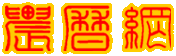回复帖子发帖表情--  作者：zhanglei1953
--

http://bbs.nongli.net/dispbbs_2_64668.html

--  作者：浪-淘-沙
--

--  作者：浪-淘-沙
--

--  作者：看不懂算法
--

--  作者：看不懂算法
--
'3、恒星时与格林尼治时间的关系 '本文所述的时间指世界时UT，世界时(0h UT)表示世界时0点,(3h UT)表示世界时3点。如果没有特另申明，均指格林尼治子午圈时间。 '格林尼治子午圈的恒星时可按如下计算: 'θo = 280.46061837 + 360.98564736629*(JD-2451545.0) + 0.000387933*T^2 - T^3/38710000 Function hxs(rlr) '计算任意时刻的格林尼治平恒星时θo sjs1 = sjs(rlr) sjs2 = sjs1 * sjs1 sjs3 = sjs2 * sjs1 hxs1 = 280.46061837 + 360.98564736629 * (rlr - 2451545#) + 0.000387933 * sjs2 - sjs3 / 38710000 hxs = jdzh(hxs1) '此时的数据是很大的度数，把±360之外的度数转换为360之内 hxs = hxs / 15 '每15度是一个小时，360/24=15 h = Int(hxs) If h < 10 Then h = "0" & h m = Int((hxs - h) * 60) If m < 10 Then m = "0" & m s = (hxs - h - (m / 60)) * 3600 If s < 1 Then s = "0" & s hxs = h & "h" & m & "m" & s End Function

--  作者：看不懂算法
--
‘2、再求出力学时TD和世界时UT的差异ΔT，'UT = TD –ΔT
Function shicha1(rlr) '计算力学时和世界时的秒差ΔT,ΔT = -15 + (JD-2382148)2/41048480
a = rlr - 2382148
b = a * a
c = 41048480
shicha1 = -15 + b / c
End Function

Function shicha2(nian) '计算力学时和世界时的秒差的另一种方法ΔT
a = nian - 1810
b = a * a
shicha2 = -15 + 0.00325 * b
End Function

Function shicha3(rlr) 'ΔT = 102.3 + 123.5T+32.5T2
a = sjs(rlr)
a2 = a * a
shicha3 = 102.3 + 123.5 * a + 32.5 * a2
End Function

‘世界时=力学时减去时差
Function ut(td, shicha1) 'UT = TD –ΔT
ut = ta - shicha1
End Function

'计算儒略世纪数T
Function sjs(rlr) '计算儒略世纪数T
sjs = (rlr - 2451545#) / 36525
End Function

--  作者：看不懂算法
--  [求助]就许建伟先生的《天文算法》一书中的疑点请教各位大侠
许建伟先生以及其同好们翻译的《天文算法》一书很好，解决了大部分业余爱好者的难题。

看了这本书，就想自己学着编写程序（自学的编写程序，不是专业出身），因为许建伟先生提供的是javascript版本的源文件，而我看不懂js语言，于是就想自己编代码来实现农历的计算功能。

在参考《天文算法》一书的过程中，问题来了。里面的知识很多，我【无法确定哪些章节】是【农历的天文计算】需要掌握的知识点。

我只想学习【农历的每年开始日期计算，农历的每月的开始日期计算，每个节气的时间计算（精确到秒%）】

若有大侠肯把那些章节指出来，不胜感激，小生在此三拜了。

下面，我就贴出自己编写的部分章节的VBA代码（可以在Excel中运行）

'VBA制作万年历的程序思路
'1、先算出儒略日JD，在【第七章】
‘A = INT（Y/100）  B = 2 - A + INT(A/4)
‘JD = INT(365.25(Y+4716))+INT(30.6001(M+1))+D+B-1524.5 （7.1）
Function rlr(nian, yue, ri, shi, fen, miao) '用年月日时分秒计算儒略日JD
a = Int(nian / 100)
b = 2 - a + Int(a / 4)
If yue = 1 Or yue = 2 Then
nian = nian - 1
yue = yue + 12
End If
fen = fen + miao / 60
shi = shi + fen / 60
ri = ri + shi / 24
rlr = Int(365.25 * (nian + 4716)) + Int(30.6001 * (yue + 1)) + ri + b - 1524.5
End Function

'''''''''''''''''''''''''以下不是必须的代码
'闰年的判断
Function rn(nian) '闰年平年的判断K
If nian Mod 400 = 0 Then
rn = 1
Else
If nian Mod 100 = 0 Then
rn = 2
Else
If nian Mod 4 = 0 Then
rn = 1
Else
rn = 2
End If
End If
End If
End Function
'星期几的判断
Function xqj(rlr) '星期几的判断
xqj = (rlr + 1.5) Mod 7
End Function
'年内序数日的判断
Function nnxsr(nian, yue, ri) '年内序数日的计算,一年内的第几日
k = rn(nian)
nnxsr = Int(275 * yue / 9) - k * Int((yue + 9) / 12) + ri - 30
End Function